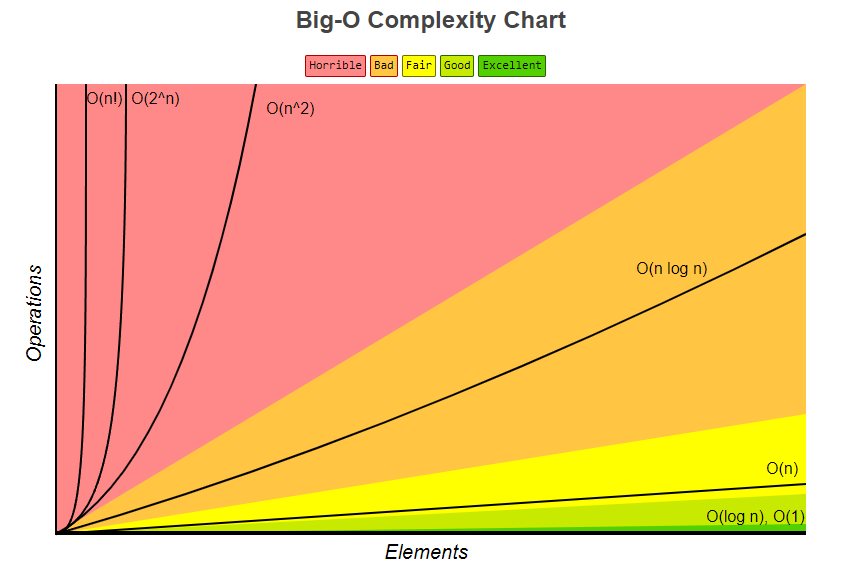# Big O Notation

“In computer science `Big O notation` is useful in the analysis of algorithms” - wikipedia.org

This illustation is from https://www.bigocheatsheet.com/ and is the most popular I’ve seen online.# Definition

• Simplified analysis of an alorithms efficiency / time complexity.

One of my peers once explained this to me as being able to use this in regards to problem solving.

Example: what’s the big O of `xs.map(...).filter(...).find(...)`

## What Is Big O?

• Complexit in terms of the input size, `n`
• Abstract the efficiency of our algorithms or code from the machine (its independent)
• Examine the basic computer steps of the code
• Can analyze both time and space

## Types of measurement

Typically look at `worst` case but we have to be cognizant of `best` and `average` case, its dependant on the application/algorithm

• Worst case (typically used)
• Best case
• Average case

## General rules

• Ignore constants for trivial examples, example a running time of `5n`, it runs on the order of `O(n)`. This is because as `n` gets large the 5 no longer matters
• in practice constants matter, a constant of 2 or 3 could have a large impact
• The same way as `n` grows certain terms `dominate` others.
• Example `O(1) < O(log n) < O(n) < O(n log n) < O(n^2) < O(2^n) < O(n!)`
• this means we ignore/drop lower order terms when dominated

### O(1) Constant time / complexity

Complexity: `Excellent`

Independent of input size `n` it takes a constant time to complete. Like 14 nanoseconds, or three minutes no matter the amount of data in the set.

Sequence of statements in constant time

### O(log n) Logarithmic time / complexity

Complexity: `Good`

“The best way to wrap your head around this is to remember the concept of halving: every time n increases by an amount k, the time or space increases by k/2.”

An example of this is binary search, see Invert A Binary Tree (BST).

### O(n) Linear time / complexity

Complexity: `Fair`

Increases linearly and in direct proportion to the number of inputs.

### O(n log n) loglinear complexity

Complexity: `Bad`

`O(n log n)` implies that `log n` operations will occur `n` times.”

### O(n^2) quadratic time / exponential time

Complexity: `Horrible`

It takes `n*n` operations, example is a nested loop.

With Big O we usually look at `worst case` scenario, for the examples below the largest runtimes are `O(n^2)`

Additionally we can have `O(nˣ)` or `polynomial complexity` which is just a range of possible values that `x` could be, generally for ccomputer science we are talking about `x` being `2`.

### O(2^n)

Complexity: `Horrible`

### O(n!) factorial

Complexity: `Horrible`

“O(n!), or factorial complexity, is the “worst” standard complexity that exists. To illustrate how fast factorial solutions will blow up in size, a standard deck of cards has 52 cards, with 52! possible orderings of cards after shuffling.”

When I was researching `Big O notation` these concepts came up a lot specifically in content from Clément Mihailescu Co-Founder of https://www.algoexpert.io/

### Sliding Window Technique

Involves manipulating two pointers or two indices at the same time as you’re traversing thought something like a string or an array. Basically you have a left pointer/index and a right pointer/index and your move them simultaneously in an array or a string. (could be to count frequency of characters)

### Recursion

A lot of problems can be solved easily with recursion (as apposed to iteratively). This produces cleaner and less code than an iterative approach. Example problems that help you understand recursion include:

• `n fibonacci`
• `calculate height of binary tree` or the `depths of nodes in a binary tree`

### Dynamic Programming (DP)

It means being able to solve a problem that is complex by first solving a smaller version of that problem. To solve that small version of the problem you solve an even smaller version of that problem, until you can solve a trivial version of the problem. The only way to get good at DP is to do a lot of DP!